• ``
• `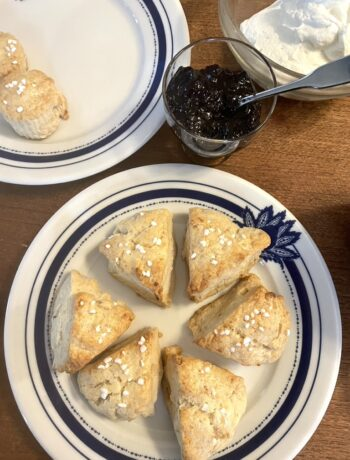`
• `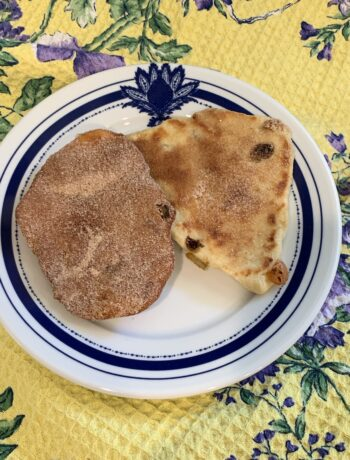`
• `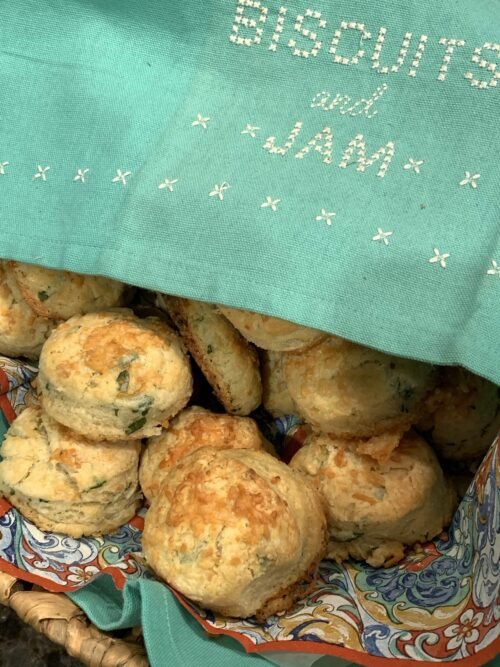`
• `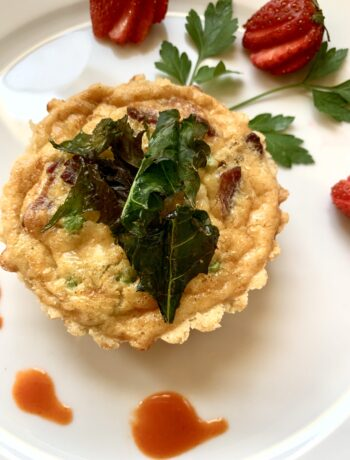`
• `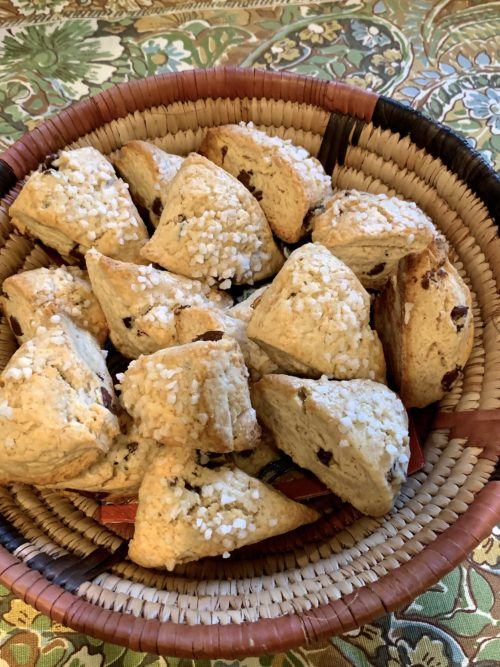`
• `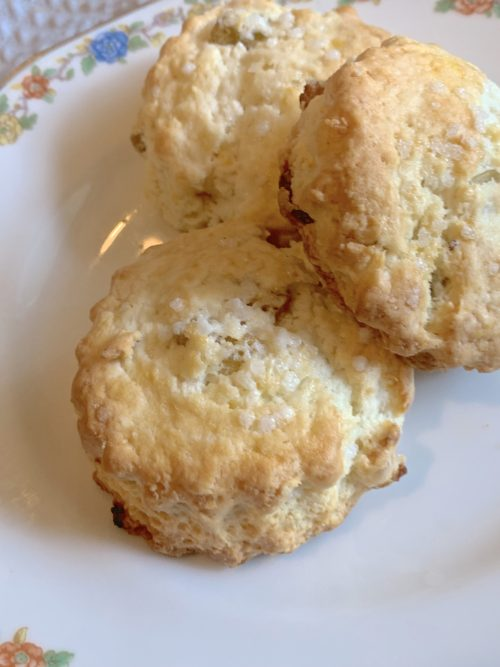`
• `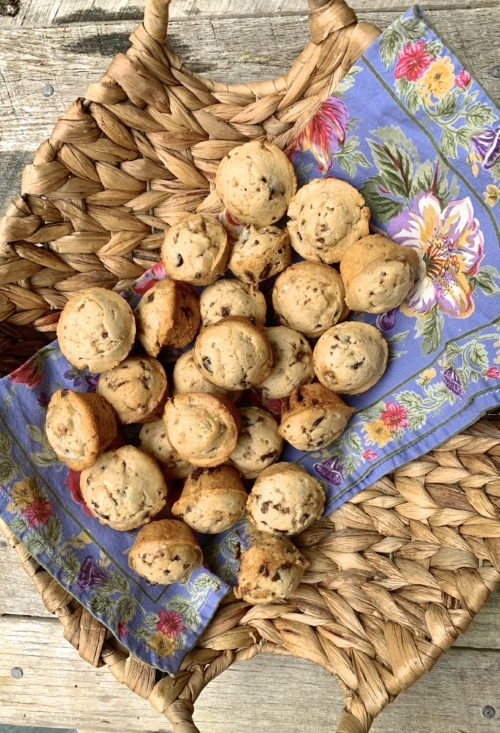`
• `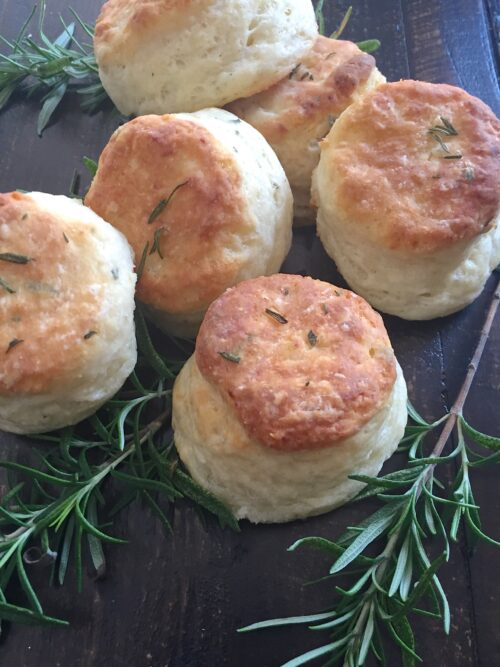`
• `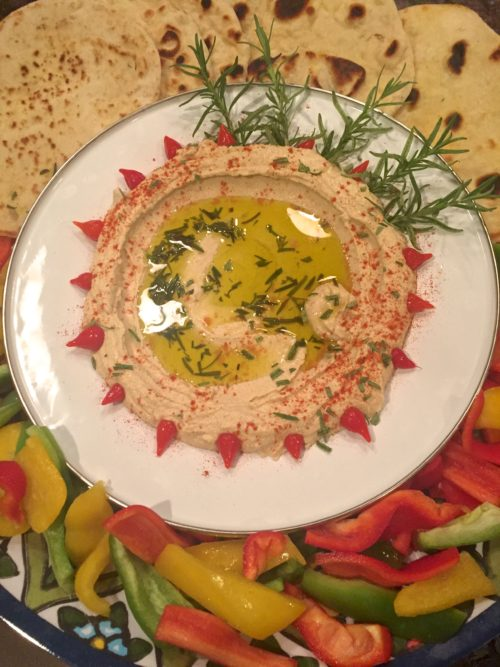`
• `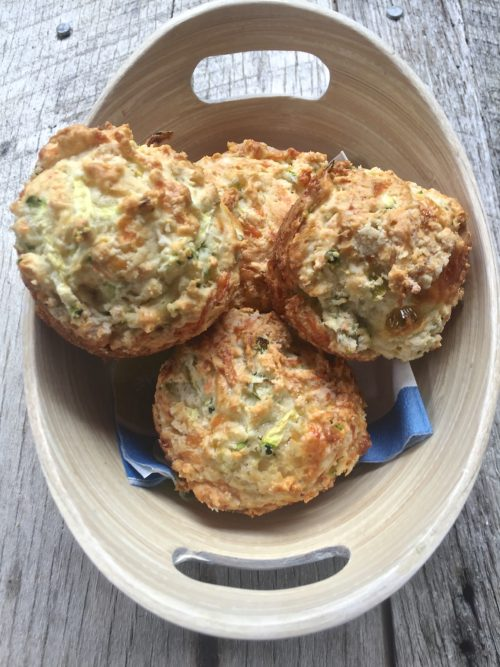`
• `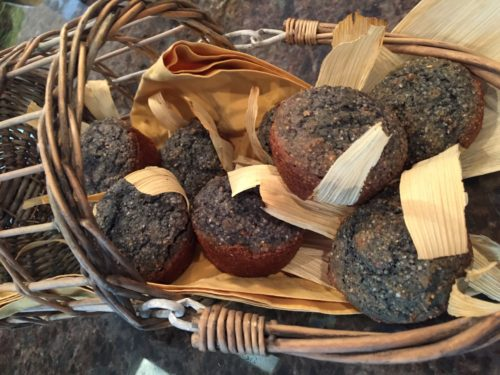`
• `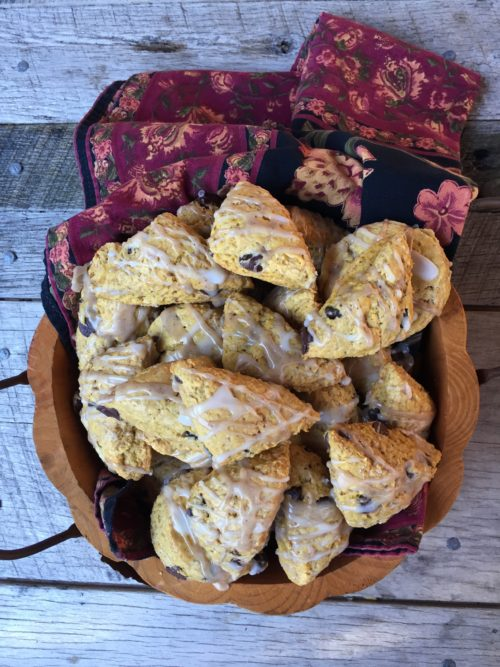`
• `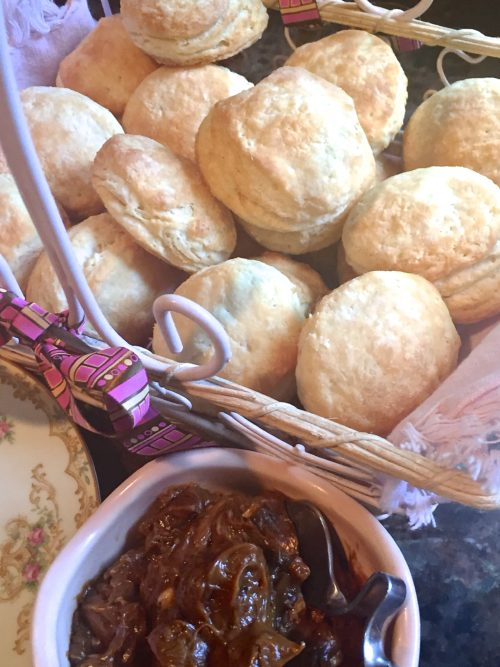`
• `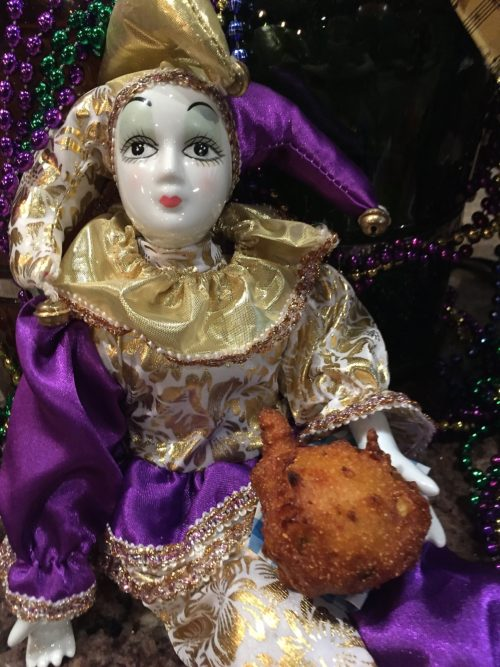`
• `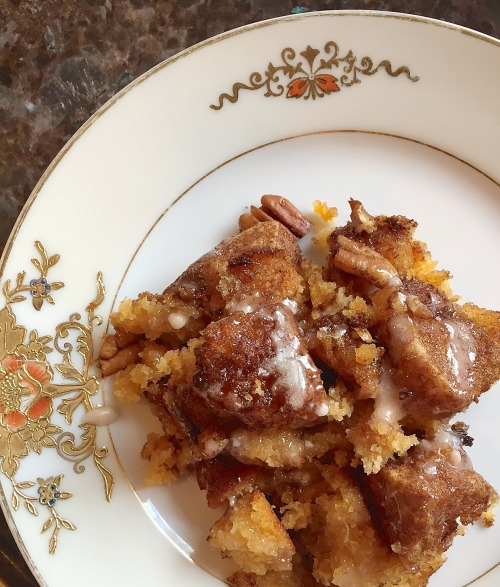`
• ``
• `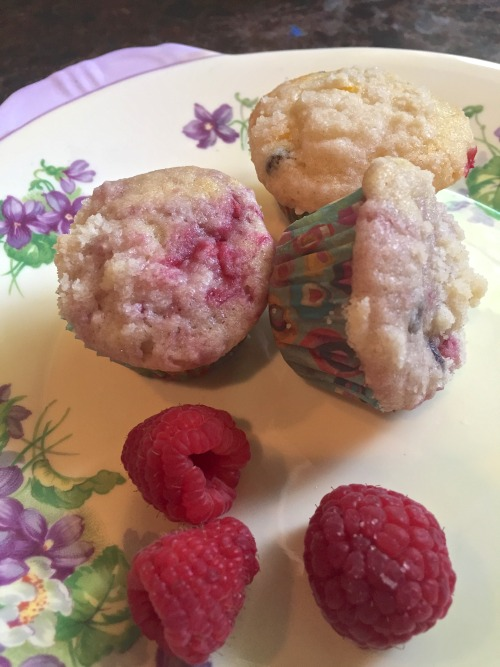`
• `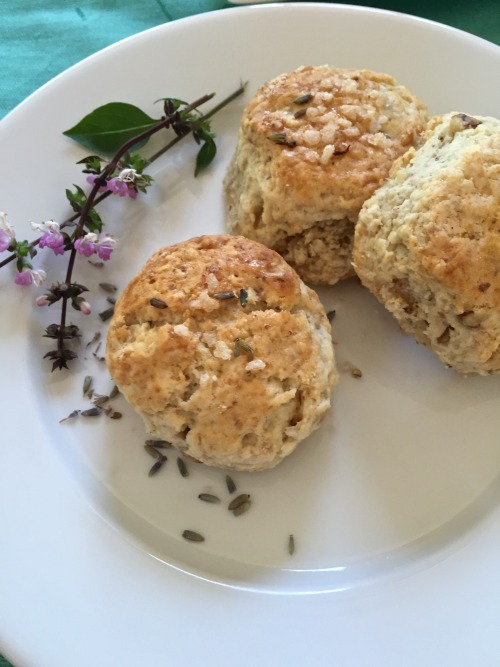`
• `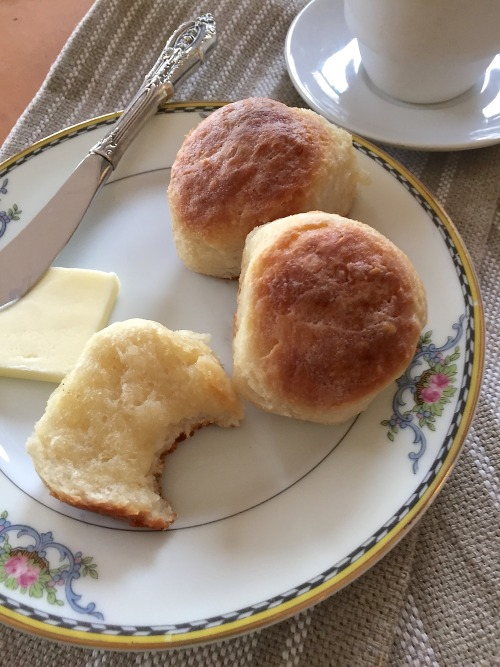`
• `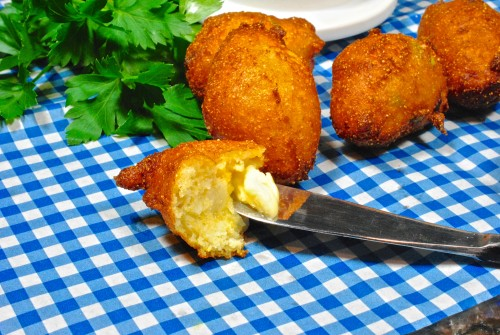`
• ``
• `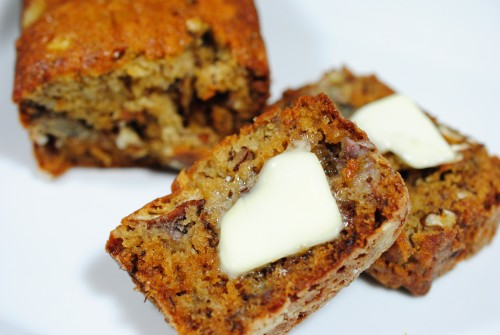`
• `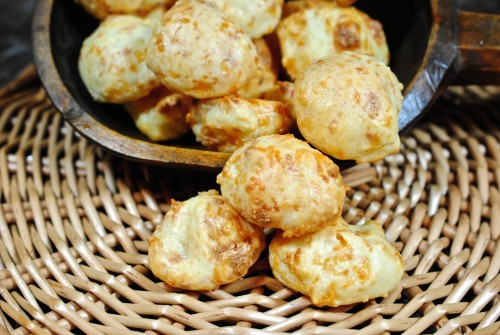`
• `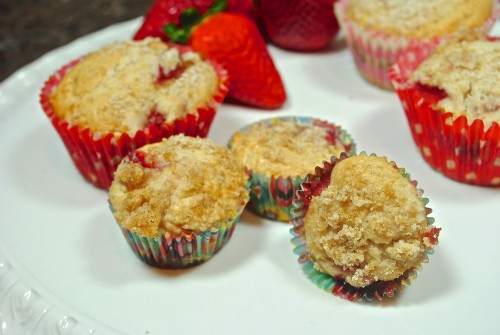`
• `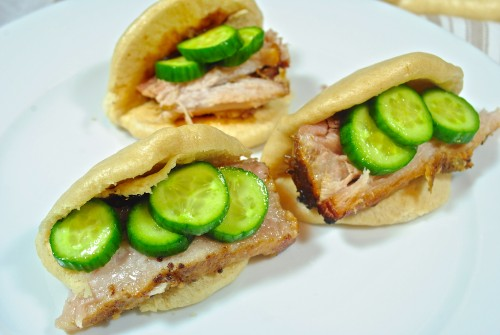`
• `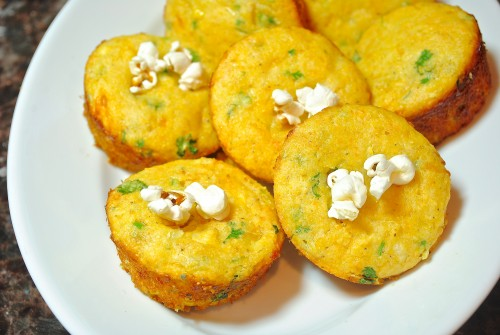`
• ``
• `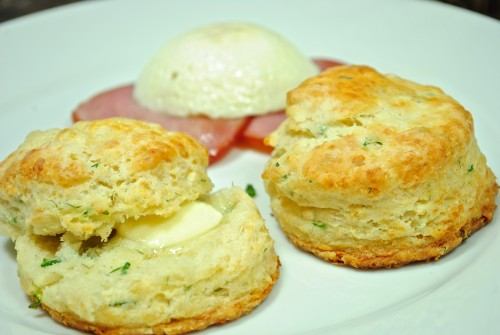`
• `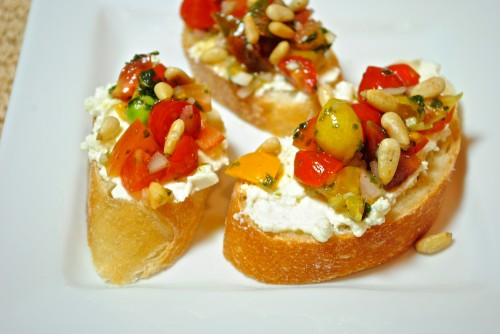`
• `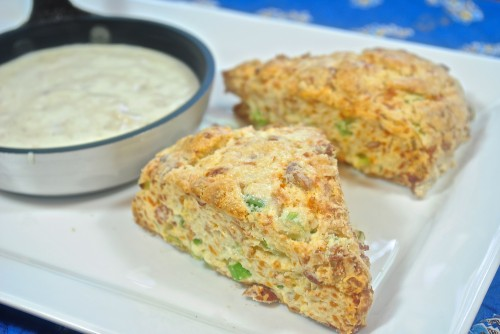`
• `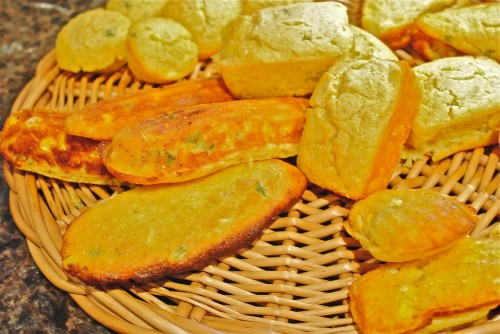`
• `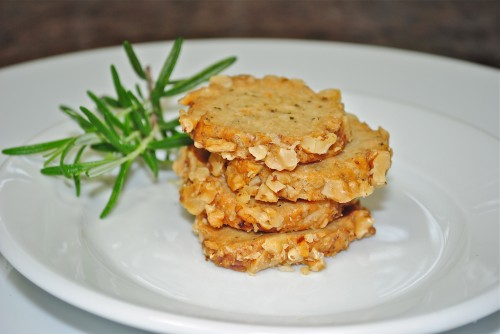`
• `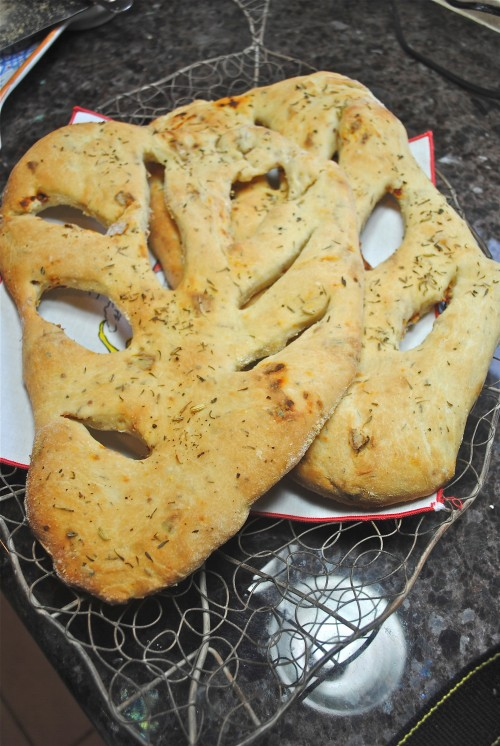`
• `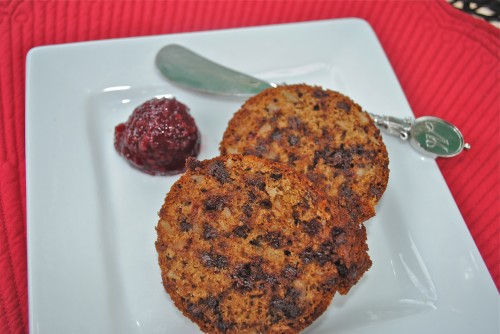`
• `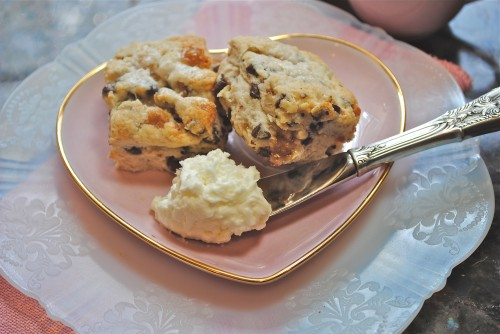`
• `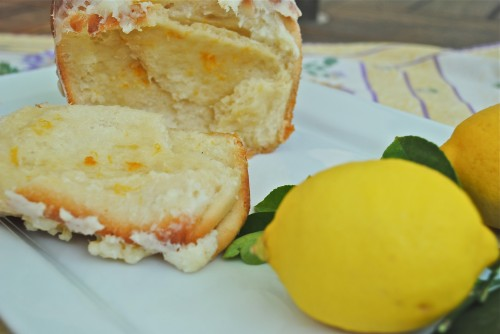`
• `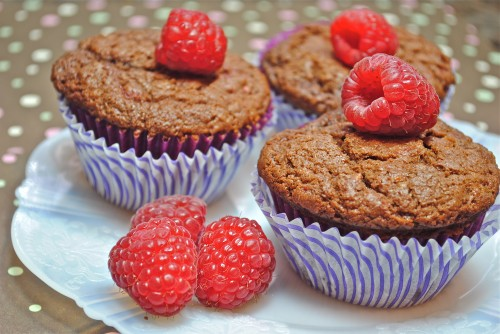`
• ``
• `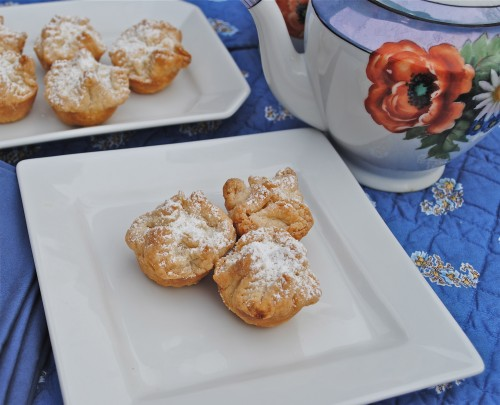`
• `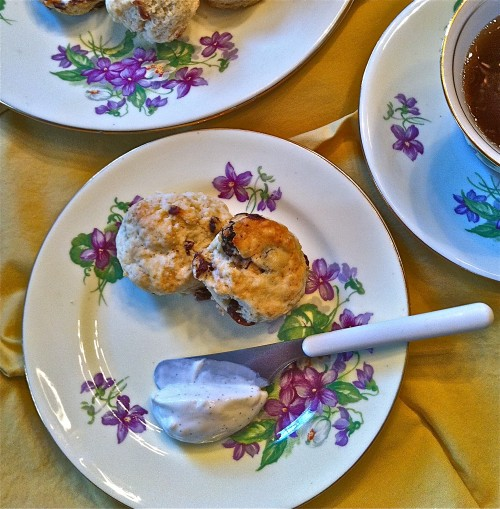`
• `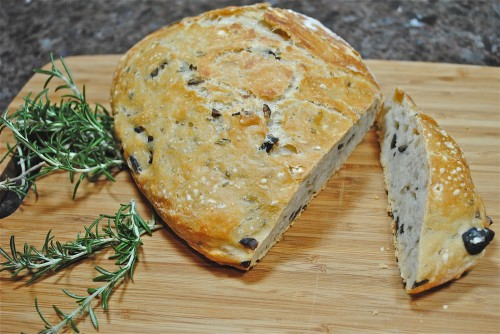`
• `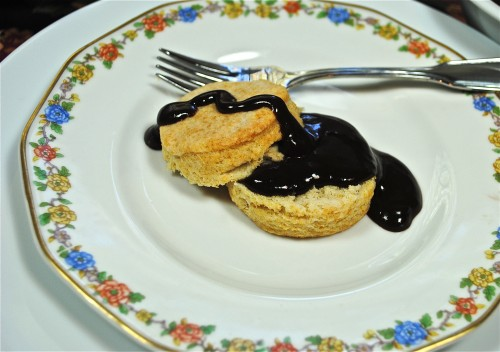`
• ``
• `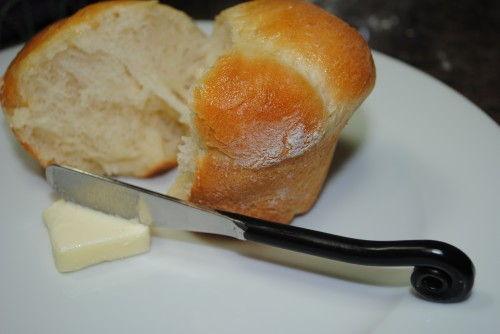`
• `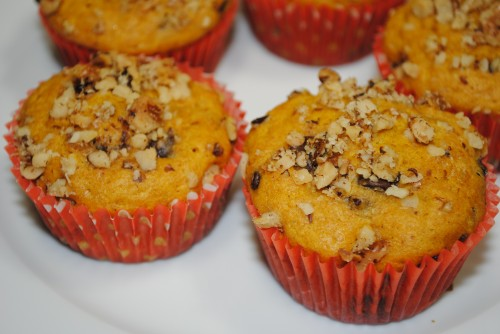`
• ``
• ``
• `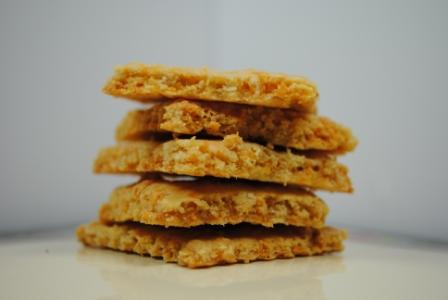`
• `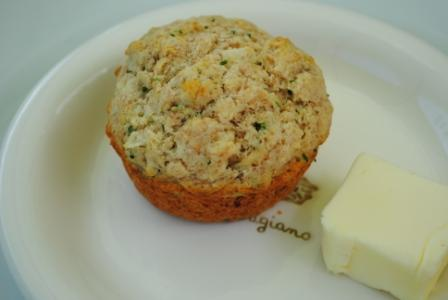`
• `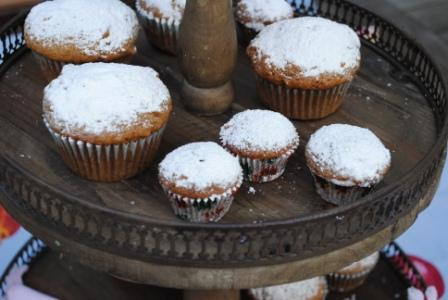`
• `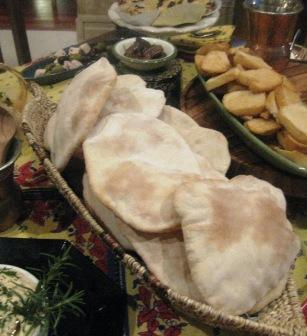`
• `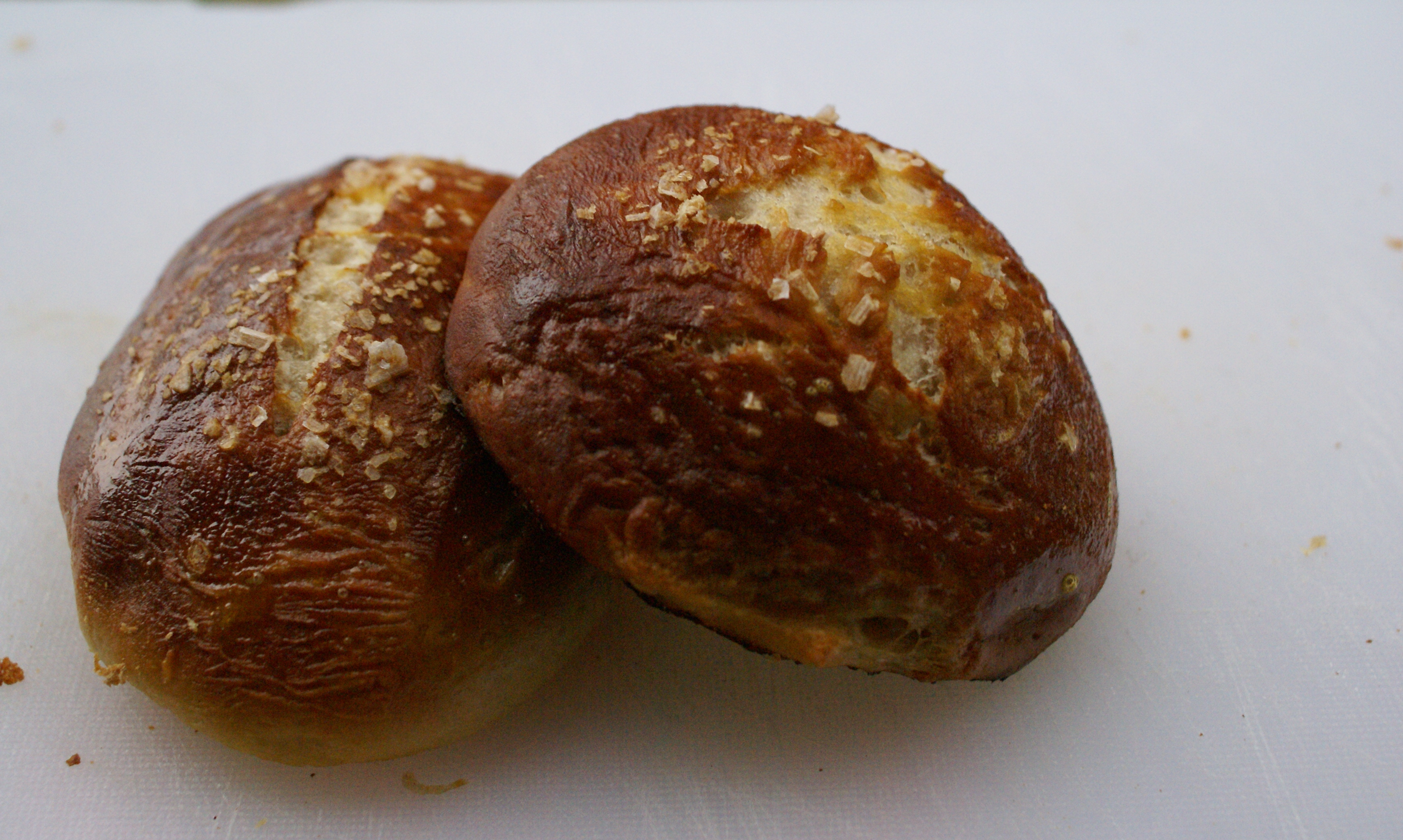`
• `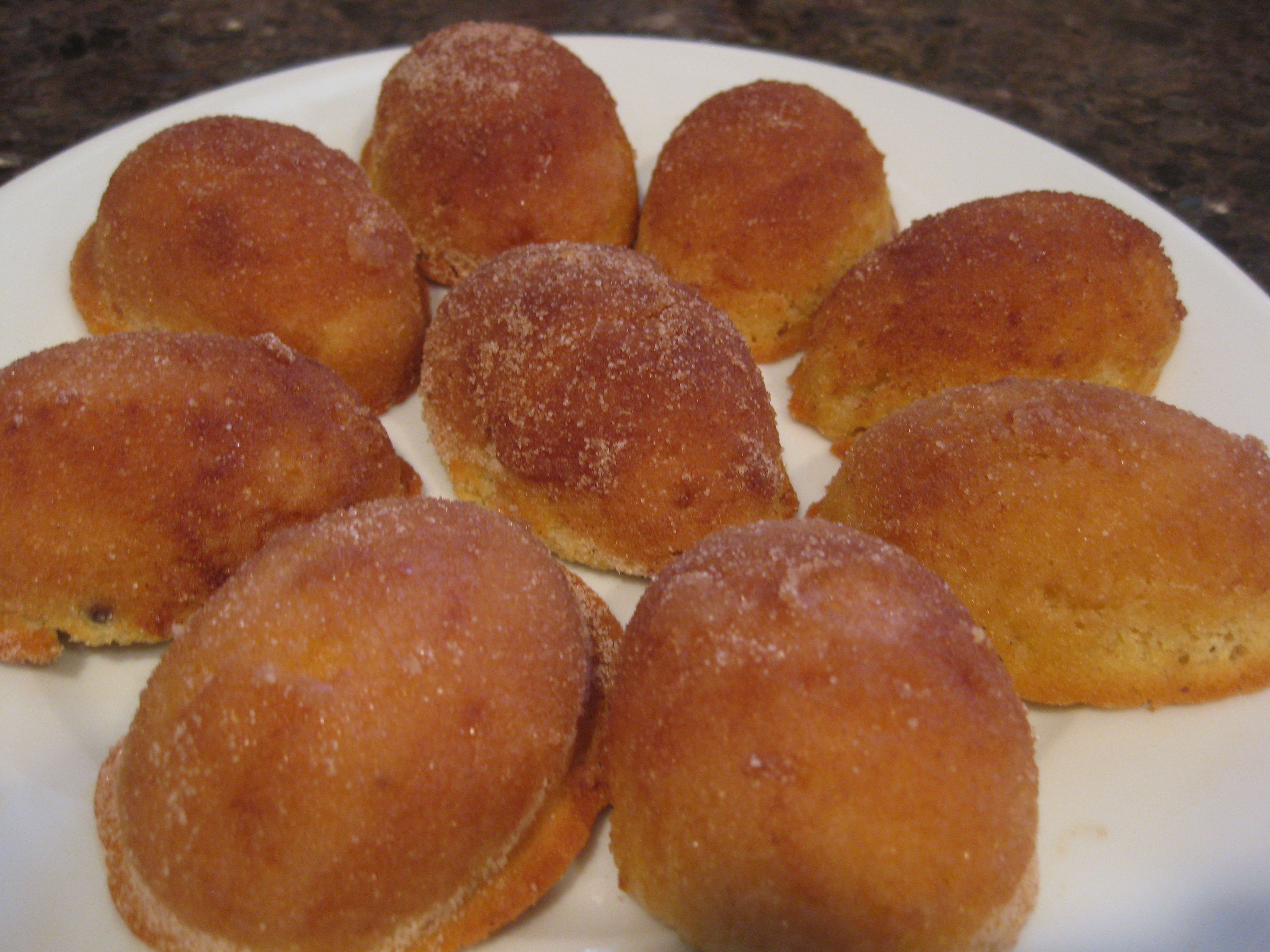`
• `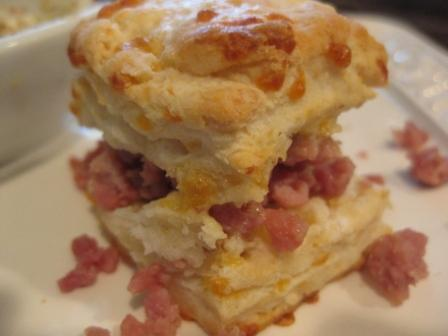`
• `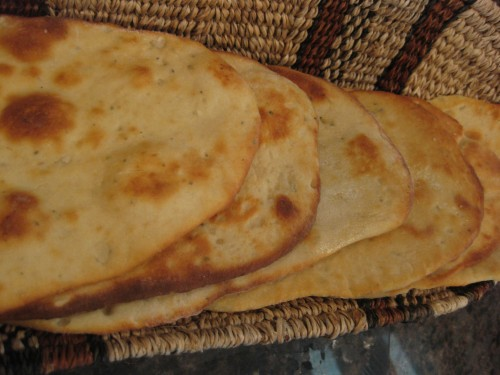`
• `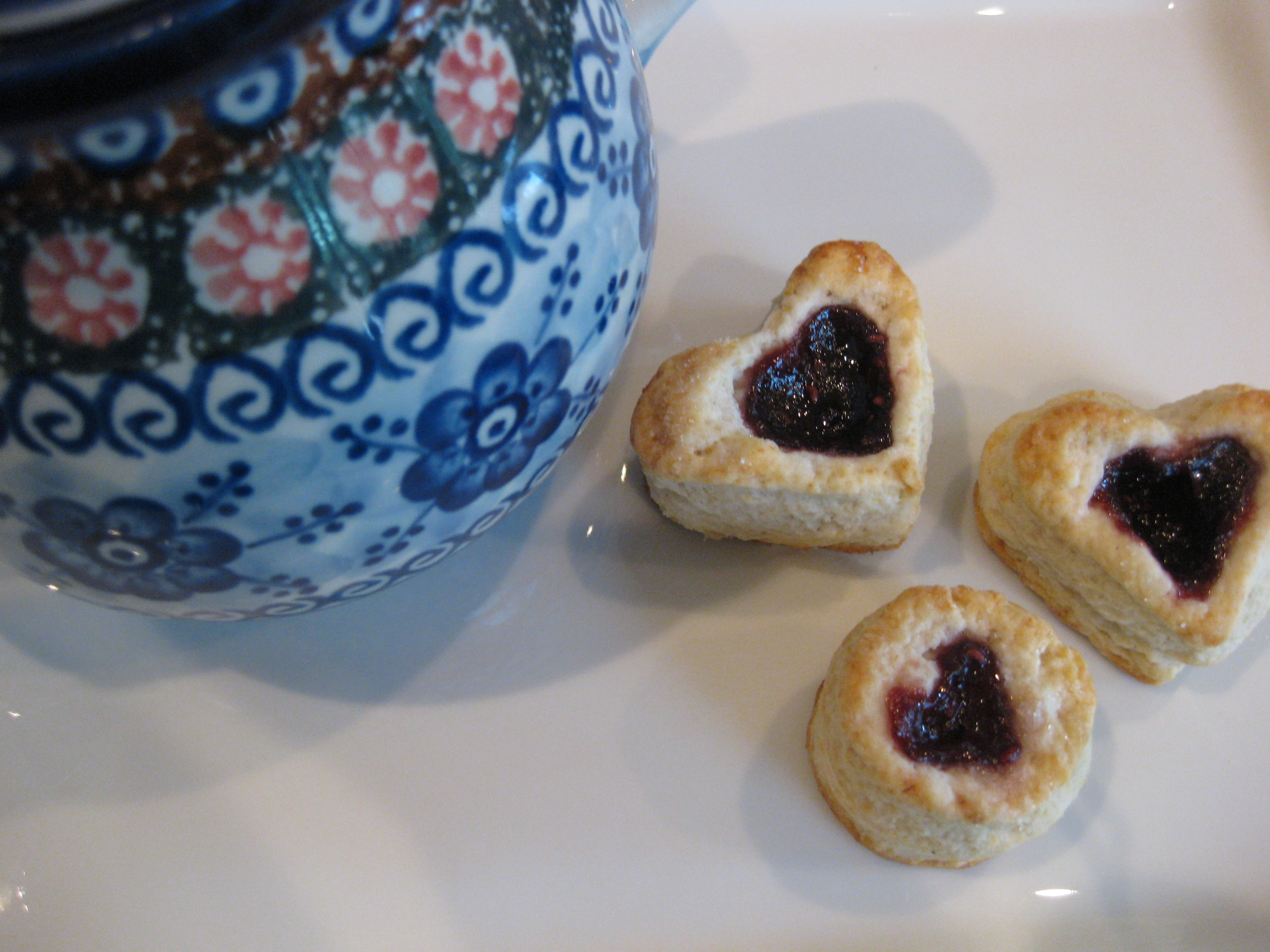`
` `